# 毕业论文案例-LDA主题模型实现文本聚类

 阿里云国际，腾讯云国际，AWS 免费开户实名账号 代冲值 优惠多多 微信号：monov8 飞机：@monov8

# LDA主题模型的预备知识

LDA主题模型本质是文本聚类算法属于文档生成模型利用样本的相似性。它常用于对文本数据的主题挖掘中比如对用户评论的挖掘人才招聘需求信息的挖掘等。它是NLP中一个常用的算法。

## 1多项式分布 Multinomial Distribution

P { X 1 = n 1 , X 2 = n 2 , … , X r = n r } = n ! n 1 ! n 2 ! … n r ! P 1 n 1 P 2 n 2 … P r n r P \lbrace X_1=n_1,X_2=n_2, \ldots ,X_r=n_r \rbrace ={n! \over n_1!n_2! \ldots n_r!} P{ ^{n_1}_1 }P{ ^{n_2}_2 } \ldots P{ ^{n_r}_r }

P 1 + P 2 + … + P r = 1 P_1+P_2+ \ldots+P_r=1

## 2狄利克雷分布 Dirichlet Distribution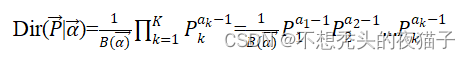# LDA主题模型的代码过程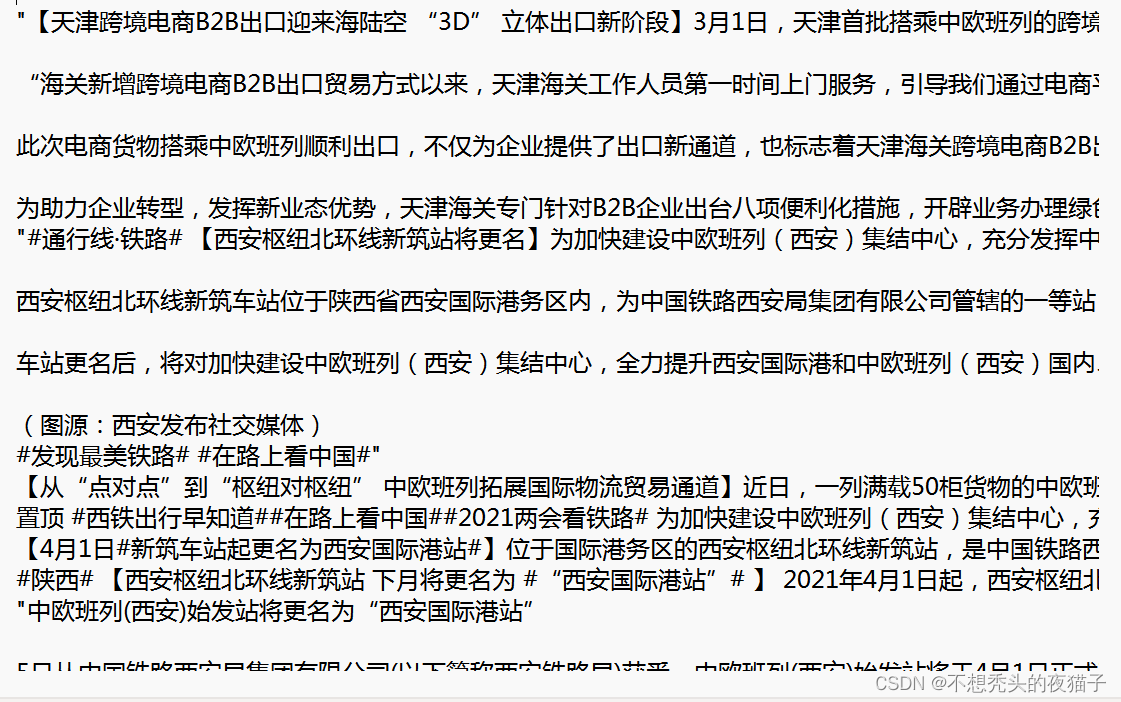## 1文本预处理

#这里定义停用词列表
def stopwordslist(filepath):
stopwords = [line.strip() for line in open(filepath, 'r', encoding='utf-8').readlines()]
return stopwords

# 对句子进行分词
def seg_sentence(sentence):
sentence = re.sub(u'[0-9\.]+', u'', sentence)

sentence_seged = jb.cut(sentence.strip())
stopwords = stopwordslist('./stopwords.txt')  # 这里加载停用词的路径
outstr = ''
for word in sentence_seged:
if word not in stopwords and word.__len__()>1:
if word != '\t':
outstr += word
outstr += " "
return outstr

#这里要注意编码问题 inputs即是读入原文本outputs即是新建一个文本然后将处理好的文本放入
inputs = open('./text.txt','r',encoding='gb18030')

outputs = open('./text03.txt','w',encoding='gb18030')
for line in inputs:
line_seg = seg_sentence(line)  # 这里的返回值是字符串
outputs.write(line_seg + '\n')
outputs.close()
inputs.close()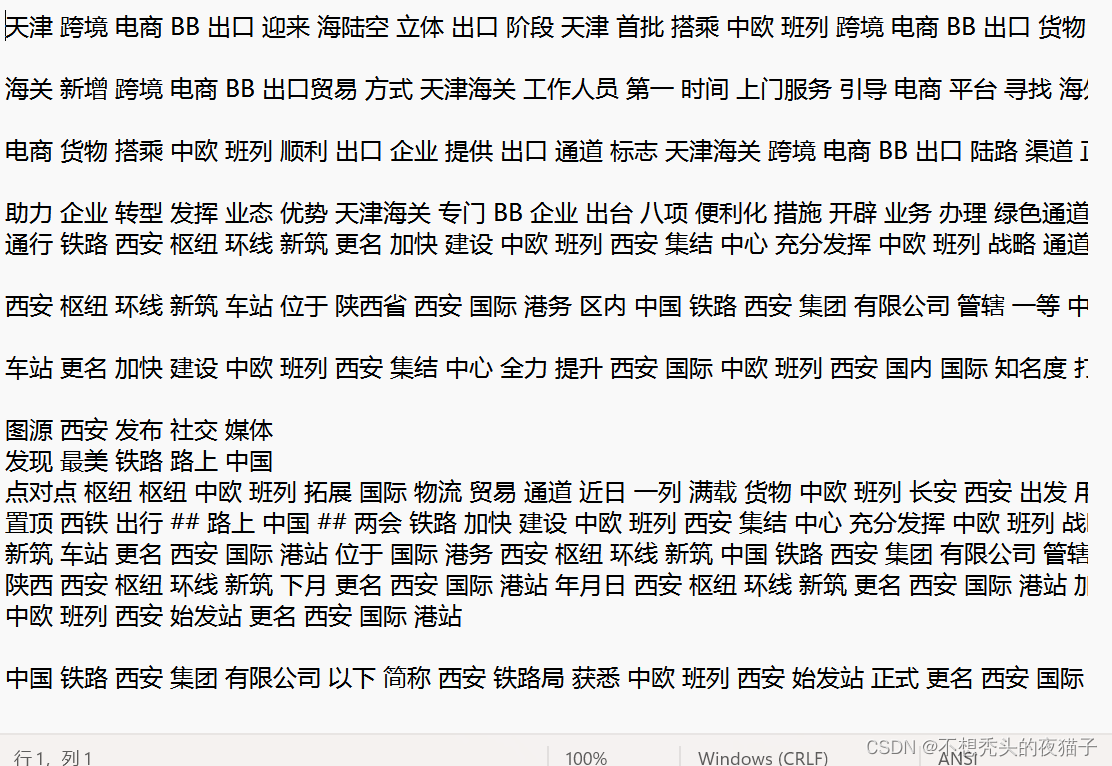## 2建模和可视化

#这里先导入常用库
from gensim import corpora
from gensim.models import LdaModel
from gensim.corpora import Dictionary
import codecs

train = []

fp.close()

'''
for line in data:
if line != '':
line = line.split(" ")
train.append([w for w in line])
'''
#以下几行将train列表的每个元素都生成一个列表 形成列表嵌套
train0=data.split(" ")
train=[]
for i in range(len(train0)):
train1=[]
train1.append(train0[i])
train.append(train1)

dictionary = corpora.Dictionary(train)
dictionary.filter_extremes(no_below=2, no_above=0.1)

corpus = [dictionary.doc2bow(text) for text in train]

lda = LdaModel(corpus=corpus, id2word=dictionary, num_topics=10, passes=50)
#corpus是词袋dictionary是词典
# num_topics主题数目
# passes训练伦次
# num_words每个主题下输出的term的数目
#corpus指语料词典

#以下为模型的输出结果即是每个主题下的20个特征词
#可以适当注释节省运行时间

for topic in lda.print_topics(num_words = 20):
termNumber = topic
print(topic, ':', sep='')
listOfTerms = topic.split('+')
for term in listOfTerms:
listItems = term.split('*')
print('  ', listItems, '(', listItems, ')', sep='')

#--------------以下是代码可视化部分------------------------------

#将可视化结果保存到lda001的网页上
import gensim
from gensim import models
import pyLDAvis.gensim_models
pyLDAvis.enable_notebook()
def lda_vis():
#corpus = gensim.corpora.MmCorpus('lda.mm')
vis = pyLDAvis.gensim_models.prepare(lda, corpus, dictionary)
return 0

if __name__ == '__main__':
lda_vis()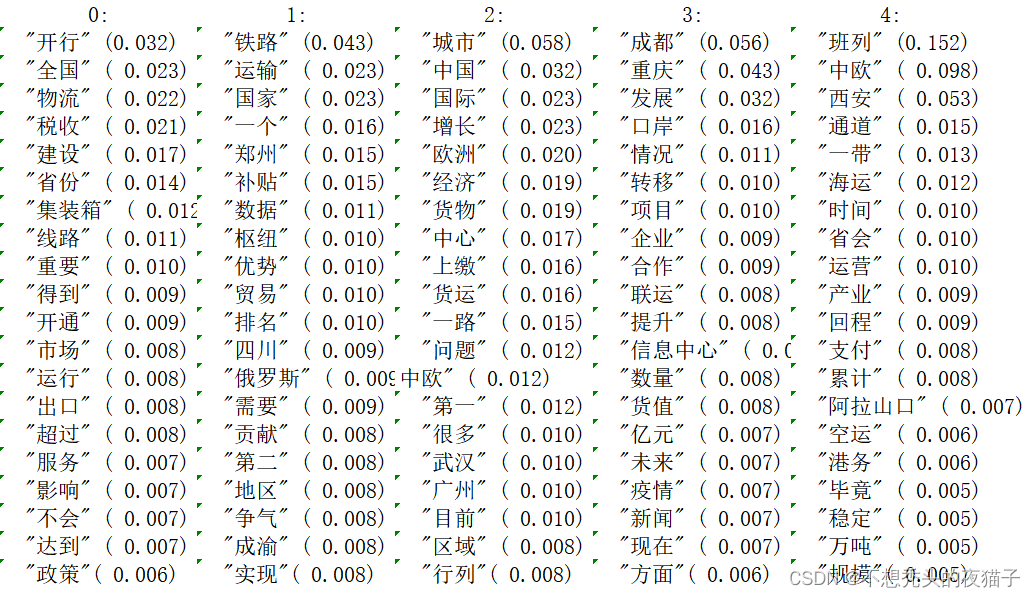1由于LDA模型是一个文档生成模型需要不断取样生成主题和词语并多次重复建模过程比较复杂运算时间比较长。因此根据情况对部分代码加上注释减少它的运行时间

2特别需要注意的是用词袋模型处理数据类型一定是一个列表嵌套对应的上文中的train是一个列表嵌套。这里大坑我花了不少时间

## 3模型优化

LDA主题模型本质上是一个文本聚类模型并且它与K-means聚类算法一样是需要我们手动输入聚类个数的。

LDA主题模型中对于主题数的选取有以下五种

#### E、主题方差

#-------------计算困惑度-------------------------------------
import codecs
import gensim
from gensim import corpora, models
import matplotlib.pyplot as plt
import matplotlib
from nltk.tokenize import RegexpTokenizer
from nltk.stem.porter import PorterStemmer

fp = codecs.open('./text03.txt','r',encoding='gb18030')
fp.close()

#以下几行将train列表的每个元素都生成一个列表 形成列表嵌套
train0=data.split(" ")
train=[]
for i in range(len(train0)):
train1=[]
train1.append(train0[i])
train.append(train1)

dictionary = corpora.Dictionary(train)  # 构建 document-term matrix
corpus = [dictionary.doc2bow(text) for text in train]
Lda = gensim.models.ldamodel.LdaModel

def perplexity(num_topics):
ldamodel = Lda(corpus, num_topics=num_topics, id2word = dictionary, passes=50)  #passes为迭代次数次数越多越精准
print(ldamodel.print_topics(num_topics=num_topics, num_words=7))  #num_words为每个主题下的词语数量
print(ldamodel.log_perplexity(corpus))
return ldamodel.log_perplexity(corpus)

# 绘制困惑度折线图
x = range(1,10)  #主题范围数量
y = [perplexity(i) for i in x]
plt.plot(x, y)
plt.xlabel('主题数目')
plt.ylabel('困惑度大小')
plt.rcParams['font.sans-serif']=['SimHei']
matplotlib.rcParams['axes.unicode_minus']=False
plt.title('主题-困惑度变化情况')
plt.show()

#----------------计算一致性-------------------------------
#计算coherence

from gensim.models.coherencemodel import CoherenceModel

def coherence(num_topics):
ldamodel = Lda(corpus, num_topics=num_topics, id2word = dictionary, passes=30,random_state = 1)
#print(ldamodel.print_topics(num_topics=num_topics, num_words=7))
ldacm = CoherenceModel(model=ldamodel, texts=train, dictionary=dictionary, coherence='c_v')
#print(ldacm.get_coherence())
return ldacm.get_coherence()

x = range(1,10)
# z = [perplexity(i) for i in x]  #如果想用困惑度就选这个
y = [coherence(i) for i in x]
plt.plot(x, y)
plt.xlabel('主题数目')
plt.ylabel('一致性大小')
plt.rcParams['font.sans-serif']=['SimHei']
matplotlib.rcParams['axes.unicode_minus']=False
plt.title('主题-一致性变化情况')
plt.show()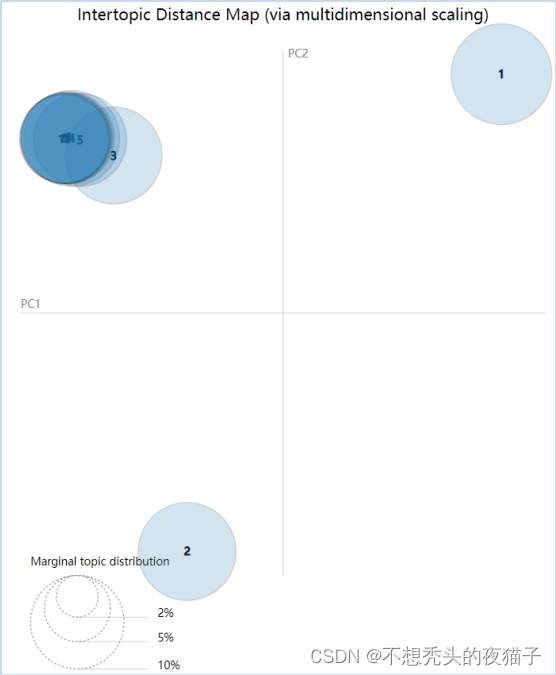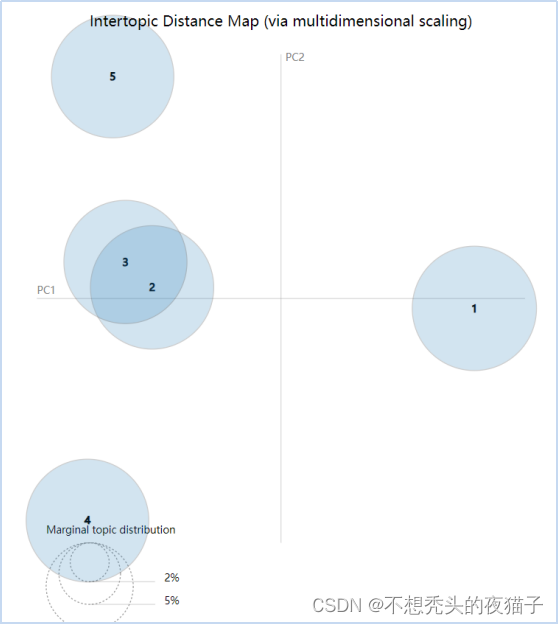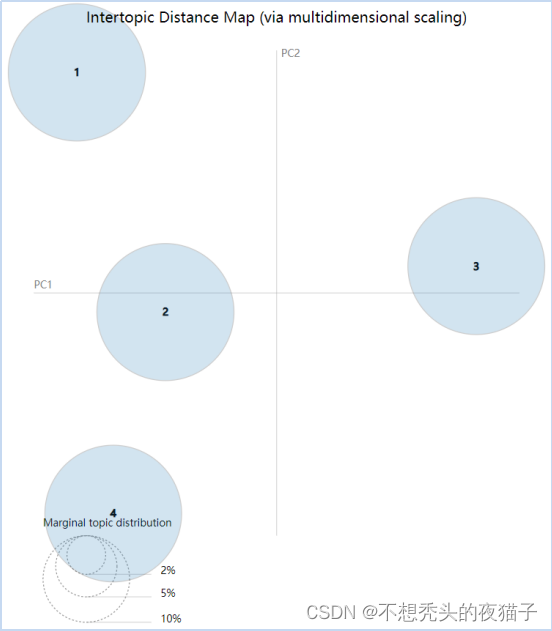# LDA主题模型的优点和不足

LDA主题模型的优点是相对于传统的主题聚类算法来说。比如PLSA算法它认为文档-主题、主题-词语的分布均是一个确定值。通俗的讲首先以一定的概率选择某个主题再在这个主题下以一定的概率选择某个词语不断的重复这两步直至生成整篇文章。

LDA主题模型的结构流程图具体可参考网上很常见这里就不再给出。

 阿里云国际，腾讯云国际，AWS 免费开户实名账号 代冲值 优惠多多 微信号：monov8 飞机：@monov8